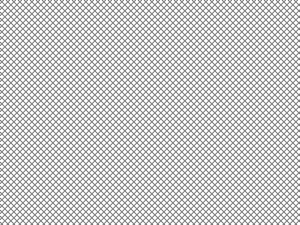# Wand Pseudo Image – Python

• Last Updated : 08 May, 2020

Wand supports a number of image format specification that is some image that are created with soem algorithms. Pseudo image is very important we can perform various manipulations to the image using Pseudo Image in wand. We can create pseudo image using setting pseudo parameter in `Image()` function.

Some manipulations we can perform –

1. Create solid color image.
3. Create images with patterns
4. Creates a fractal image.

Some important pseudo images :

Pseudo ImageDescription
‘canvas:COLOR’, or ‘xc:COLOR’Creates image with solid color, here COLOR is color value string
‘gradient:START-END’Generates a blended gradient between two colors, where both START and END are color value strings.
‘hald:’Creates a Higher And Lower Dimension matrix table.
‘inline:VALUE’where VALUE is a data-url / base64 string value..
‘label:TEXT’where TEXT is a string message.
‘pattern:LABEL’Generates image with a repeated pattern.
‘plasma:’Generates a plasma fractal image
’tile:FILENAME’Generates a repeating tile effect from a given images, where FILENAME is the path of a source image..

Syntax :

 `with Image(width``=``image_width, height``=``image_height,``                     ``pseudo``=``'pseudo_type'``) as img:``    ``# other manipulation code``    `

Now let’s get forward towards the python code for pseudo image.

Example 1: Create an image with a gradient

 `# Import Image from wand.image module``from` `wand.image ``import` `Image`` ` `# Create pseudo image using Image() function``with Image(width ``=` `400``, height ``=` `300``, ``        ``pseudo ``=``'gradient:# 32a852-# 09e846'``) as img:`` ` `    ``# Save image with a validfilename``    ``img.save(filename ``=``'gradient.png'``)`

Output :Example 2: Create an image with a pattern

 `# Import Image from wand.image module``from` `wand.image ``import` `Image`` ` `# Create image using Image() and label CROSSHATCH45 for pattern``with Image(width ``=` `100``, height ``=` `100``, pseudo ``=``'pattern:CROSSHATCH45'``) as img:`` ` `    ``# Save image``    ``img.save(filename ``=``'pattern.png'``)`My Personal Notes arrow_drop_up